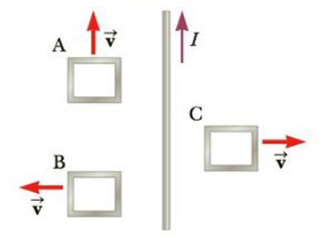Chapter 20, Problem 9P

Chapter
Section
Textbook Problem

Three loops of wire move near a long straight wire carrying a current as in Figure P20.9. What is the direction of the induced current, if any, in (a) loop A, (b) loop B, and (c) loop C.Figure P20.9

(a)

To determine
The direction of induced current, if any, in loop A.

Explanation

The loop A is moving parallel to the long straight wire carrying current. Since, the loop A is moving parallel to the long wire, there is no change in the magnetic flux in the loop A.

According to Faraday’s Law of induction, the induced emf is;

ε=ΔϕBΔt

• ΔϕB is the magnetic flux change in the loop

(b)

To determine
The direction of induced current, if any, in loop B.

(c)

To determine
The direction of induced current, if any, in loop C.

Still sussing out bartleby?

Check out a sample textbook solution.

See a sample solution

The Solution to Your Study Problems

Bartleby provides explanations to thousands of textbook problems written by our experts, many with advanced degrees!

Get Started

What are the moral implications of using ergogenic aids?

Understanding Nutrition (MindTap Course List)

Each gene is a blueprint that directs the production of one or more of the body's organs. T F

Nutrition: Concepts and Controversies - Standalone book (MindTap Course List)

What is the structure and function of the extracellular matrix?

Biology: The Dynamic Science (MindTap Course List)

What is the hybridization of carbon in

Chemistry: Principles and Reactions

How can errors in the cell cycle lead to cancer in humans?

Human Heredity: Principles and Issues (MindTap Course List)

9.13 Define the term bydrocarbon.

Chemistry for Engineering Students